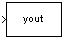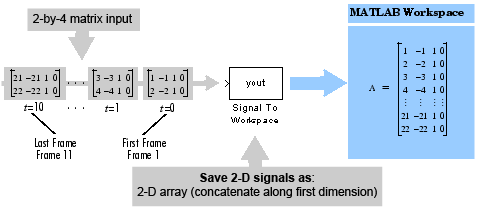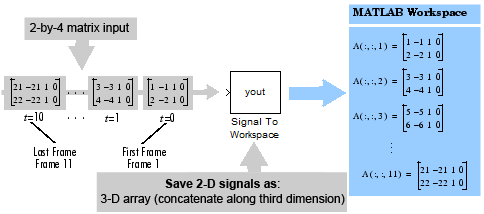# Signal To Workspace

Write data to MATLAB workspace

## Compatibility

Note

The Signal To Workspace block has been replaced by the To Workspace (Simulink) block in Simulink®. Replace existing instances of the Signal To Workspace block with To Workspace block. For new models, use the To Workspace block.

•## Library

Sinks

`dspsnks4`

## Description

The Signal To Workspace block writes data from your simulation into an array or structure in the main MATLAB® workspace. You can specify a name for the workspace variable as well as whether the data is saved as an array, structure, or structure with time.

When the Save format is set to `Array` or `Structure`, the dimensions of the output depend on the input dimensions and the setting of the Save 2-D signals as parameter. The following table summarizes the output dimensions under various conditions. In the table, K represents the value of the Limit data points to last parameter.

Input Signal DimensionsSave 2-D Signals as ...Signal To Workspace Output Dimension

M-by-N matrix

`2-D array (concatenate along first dimension)`

K-by-N matrix.

If you set the Limit data points to last parameter to `inf`, K represents the total number of samples acquired in each column by the end of simulation. This is equivalent to multiplying the input frame size (M) by the total number of M-by-N inputs acquired by the block.

M-by-N matrix

`3-D array (concatenate along third dimension)`

M-by-N-by-K array.

If you set the Limit data points to last parameter to `inf`, K represents the total number of M-by-N inputs acquired by the end of the simulation.

Length-N unoriented vector

Any setting

K-by-N matrix

N-dimensional array where N > 2

Any setting

Array with N+1 dimensions, where the size of the last dimension is equal to K. If you set the Limit data points to last parameter to `inf`, K represents the total number of M-by-N inputs acquired by the end of simulation

## Examples

### Example 1: Save 2-D Signals as a 2-D Array

In the ex_signaltoworkspace_ref2 model, the Signal To Workspace block receives a 2-by-4 matrix input and logs 11 frames (two samples per frame) by the end of the simulation. Because the Save 2-D signals as parameter is set to ```2-D array (concatenate along first dimension)```, the block concatenates the input along the first dimension to create a 22-by-4 matrix, A, in the MATLAB workspace.

The following figure illustrates the behavior of the Signal to Workspace block in this example.In the 2-D output mode, there is no indication of where one frame ends and another begins. To log input frames separately, set the Save 2-D signals as parameter to `3-D array (concatenate along third dimension)`, as shown in Example 2.

### Example 2: Save 2-D Signals as a 3-D Array

In the ex_signaltoworkspace_ref1 model, the input to the Signal To Workspace block is a 2-by-4 matrix. The Save 2-D signals as parameter is set to ```3-D array (concatenate along third dimension)```, so by the end of the simulation the Signal To Workspace block logs 11 frames of data as a 2-by-4-by-11 array, A, in the MATLAB workspace.

The following figure illustrates the behavior of the Signal to Workspace block in this example.## Parameters

Variable name

Specify the name of the array or structure into which the block logs the simulation data. The block creates this variable in the MATLAB workspace only after the simulation stops running. When you enter the name of an existing workspace variable, the block overwrites that variable with the simulation data.

Limit data points to last

Specify the maximum number of samples or frames the block will save. When the simulation generates more than the specified maximum number of samples or frames, the simulation saves only the most recently generated data. To capture all data, set this parameter to `inf`. See the table in the Description section for more information on how this parameter affects the dimensions of the logged data.

Decimation

Specify a positive integer d to determine how often the block writes data to the workspace array or structure. The block writes data to the array or structure every dth sample. With the default decimation value of `1`, the block writes data at every time step.

Save format

Specify the format in which to save simulation output to the workspace. You can select one of the following options:

• `Array` — Select this option to save the data as an N-dimensional array. If the input signal is an unoriented vector, the resulting workspace array is 2-D. Each input vector is saved in a row of the output matrix, vertically concatenated onto the previous vector. If the input signal is 2-dimensional, the dimensions of the resulting workspace array depend on the setting of the Save 2-D signals as parameter.

• `Structure` — Select this option to save the data as a structure consisting of three fields: `time`, `signals` and `blockName`. In this mode, the `time` field is empty, and the `blockName` field contains the name of the Signal To Workspace block. The `signals` field contains a structure with three additional fields: `values`, `dimensions`, and `label`. The `values` field contains the array of signal values, the `dimensions` field specifies the dimensions of the values array, and the `label` field contains the label of the input line.

• `Structure with time` — This option is the same as `Structure`, except that the `time` field contains a vector of simulation time steps. This is the only output format that can be read directly by a From Workspace block. When you select this option, the Save 2-D signals as parameter is not available. In this mode, the block always saves 2-D input arrays as a 3-D array.

The default setting of this parameter is `Array`.

Save 2-D signals as

Specify whether the block outputs 2-D signals as a 2-D or 3-D array in the MATLAB workspace:

• `2-D array (concatenate along first dimension)` — When you select this option, the block saves an M-by-N input signal as a (K*M)-by-N matrix, where K*M is the total number of samples acquired by the end of the simulation. The block vertically concatenates each M-by-N matrix input with the previous input to produce the 2-D output array. See Example 1: Save 2-D Signals as a 2-D Array for more information about this mode.

• `3-D array (concatenate along third dimension)` — When you select this option, the block saves an M-by-N input signal as an M-by-N-by-K array, where K is the number of M-by-N inputs logged by the end of the simulation. K has an upper bound equal to the value of the Limit data points to last parameter. See Example 2: Save 2-D Signals as a 3-D Array for more information about this mode.

This parameter is visible only when you set the Save format parameter to `Array` or `Structure`. When you set the Save format parameter to ```Structure with time```, the block outputs the 2-D input signal as a 3-D array.

Note

The ```Inherit from input (this choice will be removed - see release notes)``` option will be removed in a future release. See Signal To Workspace Block Changes in the DSP System Toolbox™ Release Notes for more information.

Log fixed-point data as a fi object

Select this check box to log fixed-point data to the MATLAB workspace as a Fixed-Point Designer™ `fi` object. Otherwise, fixed-point data is logged to the workspace as `double`.

## Supported Data Types

• Double-precision floating point

• Single-precision floating point

• Fixed point (signed and unsigned)

• 8-, 16-, and 32-bit signed integers

• 8-, 16-, and 32-bit unsigned integers